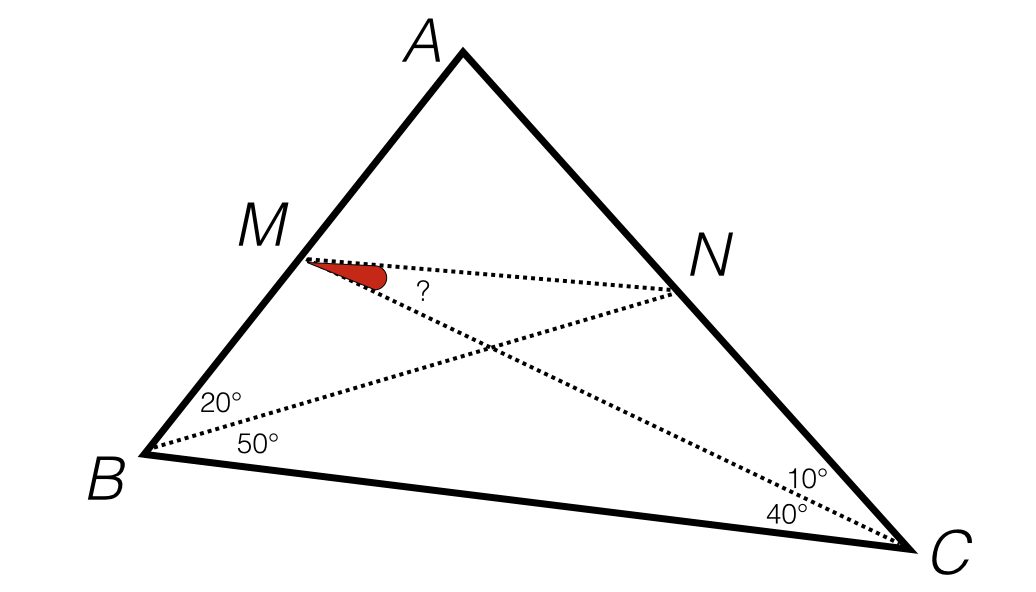# Is Angle Chasing Useful?

Geometry Level 5In triangle $ABC$, $\angle{B} = 70^{\circ}$ and $\angle{C} = 50^{\circ}$. Point $M$ is placed on side $AB$ such that $\angle{MCB} = 40^{\circ}$ and point $N$ is placed on side $AC$ such that $\angle{NBC} = 50^{\circ}$.

Find $\angle{NMC}$ (in degrees).

×

Problem Loading...

Note Loading...

Set Loading...